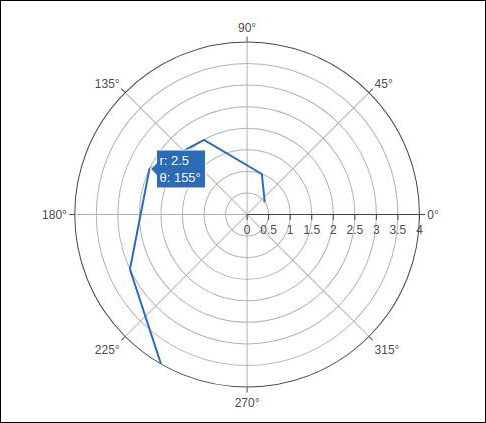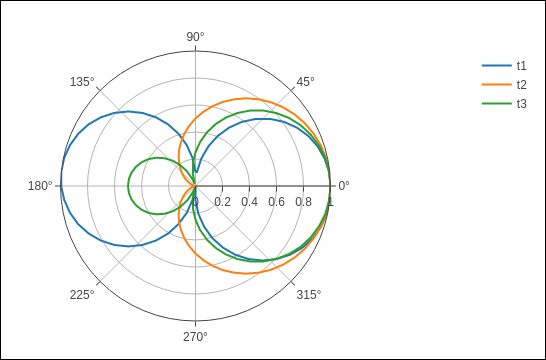# Plotly - Polar Chart and Radar Chart

In this chapter, we will learn how Polar Chart and Radar Chart can be made with the help Plotly.

First of all, let us study about polar chart.

## Polar Chart

Polar Chart is a common variation of circular graphs. It is useful when relationships between data points can be visualized most easily in terms of radiuses and angles.

In Polar Charts, a series is represented by a closed curve that connect points in the polar coordinate system. Each data point is determined by the distance from the pole (the radial coordinate) and the angle from the fixed direction (the angular coordinate).

A polar chart represents data along radial and angular axes. The radial and angular coordinates are given with the r and theta arguments for go.Scatterpolar() function. The theta data can be categorical, but, numerical data are possible too and is the most commonly used.

Following code produces a basic polar chart. In addition to r and theta arguments, we set mode to lines (it can be set to markers well in which case only the data points will be displayed).

import numpy as np
r1 = [0,6,12,18,24,30,36,42,48,54,60]
t1 = [1,0.995,0.978,0.951,0.914,0.866,0.809,0.743,0.669,0.588,0.5]
trace = go.Scatterpolar(
r = [0.5,1,2,2.5,3,4],
theta = [35,70,120,155,205,240],
mode = 'lines',
)
data = [trace]
fig = go.Figure(data = data)
iplot(fig)


The output is given below −In the following example data from a comma-separated values (CSV) file is used to generate polar chart. First few rows of polar.csv are as follows −

y,x1,x2,x3,x4,x5,
0,1,1,1,1,1,
6,0.995,0.997,0.996,0.998,0.997,
12,0.978,0.989,0.984,0.993,0.986,
18,0.951,0.976,0.963,0.985,0.969,
24,0.914,0.957,0.935,0.974,0.946,
30,0.866,0.933,0.9,0.96,0.916,
36,0.809,0.905,0.857,0.943,0.88,
42,0.743,0.872,0.807,0.923,0.838,
48,0.669,0.835,0.752,0.901,0.792,
54,0.588,0.794,0.691,0.876,0.74,
60,0.5,0.75,0.625,0.85,0.685,


Enter the following script in notebook’s input cell to generate polar chart as below −

import pandas as pd
t1 = go.Scatterpolar(
r = df['x1'], theta = df['y'], mode = 'lines', name = 't1'
)
t2 = go.Scatterpolar(
r = df['x2'], theta = df['y'], mode = 'lines', name = 't2'
)
t3 = go.Scatterpolar(
r = df['x3'], theta = df['y'], mode = 'lines', name = 't3'
)
data = [t1,t2,t3]
fig = go.Figure(data = data)
iplot(fig)


Given below is the output of the above mentioned code −A Radar Chart (also known as a spider plot or star plot) displays multivariate data in the form of a two-dimensional chart of quantitative variables represented on axes originating from the center. The relative position and angle of the axes is typically uninformative.

For a Radar Chart, use a polar chart with categorical angular variables in go.Scatterpolar() function in the general case.

Following code renders a basic radar chart with Scatterpolar() function

radar = go.Scatterpolar(
r = [1, 5, 2, 2, 3],
theta = [
'processing cost',
'mechanical properties',
'chemical stability',
'thermal stability',
'device integration'
],
fill = 'toself'
)